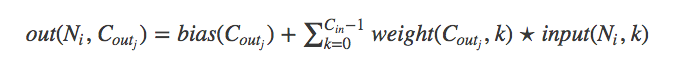# What is an example of a 1D convolution that has more than 1 input channel?

I was reading http://pytorch.org/docs/master/nn.html#conv1d to understand 1D convolutions and I was trying to understand when would the `in_channels` not equal to 1.

If we have say a 1D array of size `1 by D` and we want to convolv it with a `F=3` filters of size `K=2` say and not skipping any value (i.e. stride=1), would the code be:

``````F,K=3,2
m = nn.Conv1d(1, F, K, stride=1)
``````

I am just not sure when the `in_channels` would not be 1 for a 1D convolution. Furthermore, assuming it is possible for it to not be 1, does it mean when its not one? Is it not 1 when there are lots of 1D strips we are considering, say in a data set of size `N` (though not sure what that type of data set would mean…)

2 Likes

You could stack different time signals together and thus create one time signal with multiple channels. Imaging EEG data filtered with different bandpasses. You could create a signal for each band, concat these signals and convolve through the time dimension using all input channels.
It’s comparable to the color channels of an image.
I hope it makes sense to you5 Likes

if one did that would `nn.Conv1d(1, F, K, stride=1)` combine the output of each filer? I guess it would based on the equation:which I find super strange. What would the meaning of combining the processing of one data point to another? Isn’t that weird? Maybe its not but I don’t understand the motivation to do it.

Each filter in the conv layer would convolve over the input signal using its kernel size F and all input channels.
Assuming the kernel size is 3 and the input signal has 5 channels, each filter will have 3*5=15 weights and a bias.
I don’t really understand your question regarding the processing of one data point to another.

Another example could be temperature and humidity measurements.
e.g.
at 9am: temp 10°, humidity 60%
at 10am: temp 13°, humidity 57%

Each point in time would have two values. In this example the input data has two channels.

With kernel 2 and stride 1, the convolution will look at successive pairs of timestep, looking at two values for each timestep because there are two channels.

If you specify 3 output channels then the conv1d will be applied 3 times to the input with 3 different sets of weights, and produce 3 output values per timestep.

I hope this is becoming clearer.

3 Likes

Stereo audio when you don’t want to sum the channels. There has been some good results with neural networks that use the two channels (microphones setup in different parts of the room) to determine background noise vs speech and filter it out.